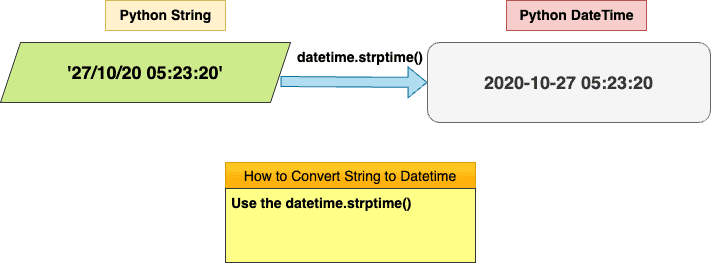# How to Convert String to Datetime in PythonTo convert a string to a datetime object in Python, you can use the “datetime.strptime()” method from the datetime module. The strptime() method takes an argument where you may provide date_string and format and returns the string representation of the​ date/time to a datetime object.

## Syntax

``datetime.strptime(date_string, format)``

## Parameters

1. date_string: It is a date string.
2. format: It is an output format for datetime.

## Example 1: Using the strptime() method

``````from datetime import datetime

dt_str = '27/10/20 05:23:20'

dt_obj = datetime.strptime(dt_str, '%d/%m/%y %H:%M:%S')

print("The type of the date is now", type(dt_obj))
print("The date is", dt_obj)``````

Output

``````The type of the date is now <class 'datetime.datetime'>
The date is 2020-10-27 05:23:20``````

The datetime.strptime() is a general method for parsing strings into datetimes. It can handle all sorts of formats, with the format defined by the format string you give.

## Example 2: Converting string to datetime using dateutil

The dateutil library provides a parser that can be used to convert strings to datetime objects. The dateutil.parser.parse() function takes a string representing a date and time and returns a datetime object.

The dateutil can be installed from PyPI using the pip package manager.

Import the dateutil package and use the parser module to convert the string to datetime.

``````from dateutil import parser

dt_str = '27/10/20 05:23:20'

dt_obj = parser.parse(dt_str)

print("The type of the date is now", type(dt_obj))
print("The date is", dt_obj)``````

Output

``````The type of the date is now <class 'datetime.datetime'>
The date is 2020-10-27 05:23:20``````

## Example 3: Converting a String to various datetime objects

### Example 3.1: Converting a String to datetime.datetime() Object

Use the datetime.strptime() method to convert a string to datetime.datetime() object in Python.

``````from datetime import datetime

date_string = "2023-01-20 19:27:56"
datetime_object = datetime.strptime(date_string, "%Y-%m-%d %H:%M:%S")
print(datetime_object)``````

#### Output

``2023-01-20 19:27:56``

### Example 3.2: Converting a String to datetime.date() Object

To convert a string to datetime.date() object, use the datetime.strptime() method and apply the .date() function on a result of the .strptime() function.

``````from datetime import datetime

date_string = "2023-01-20"
date_object = datetime.strptime(date_string, "%Y-%m-%d").date()
print(date_object)``````

Output

``2023-01-20``

### Example 3.3: Converting a String to datetime.time() Object

To convert a string to datetime.time() object, use the datetime.strptime() method and apply the .time() function on a result of the .strptime() function.

``````from datetime import datetime

time_string = "19:39:50"
time_object = datetime.strptime(time_string, "%H:%M:%S").time()
print(time_object)``````

Output

``19:39:50``

### Example 3.4: Converting String to datetime.datetime() Object with Locale

To set a locale in Python, import the locale package in your program and then use the locale.setlocale() method to set the locale.

``````import locale
from datetime import datetime

locale.setlocale(locale.LC_ALL, 'es_ES')
date_str_es = '27-Octubre-2020' # es_ES locale
datetime_object = datetime.strptime(date_str_es, '%d-%B-%Y')
print(datetime_object)``````

Output

``2020-10-27 00:00:00``

That’s it.

## Related posts

Python datetime to string

Python Date Format

This site uses Akismet to reduce spam. Learn how your comment data is processed.